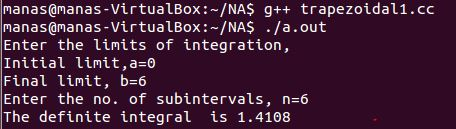# C++ Program for Trapezoidal Rule for the Evaluation of Definite Integrals

```//Trapezoidal Method for the evaluation of Definite Integrals
#include<iostream>
#include<cmath>
using namespace std;
double f(double x)        //write the function whose definite integral is to be calcuated here
{
double a=1/(1+x*x);
return a;
}
int main()
{
int n,i;        //n is for subintervals and i is for loop
double a,b,h,sum=0,integral;
cout<<"Enter the limits of integration,\nInitial limit,a=";    //get the limits of integration
cin>>a;
cout<<"Final limit, b=";
cin>>b;
cout<<"Enter the no. of subintervals, n=";            //get the no. of subintervals
cin>>n;
double x[n+1],y[n+1];
h=(b-a)/n;                //get the width of the subintervals
for (i=0;i<=n;i++)
{                    //loop to evaluate x0,...xn and y0,...yn
x[i]=a+i*h;            //and store them in arrays
y[i]=f(x[i]);
}
for (i=1;i<n;i++)            //loop to evaluate h*(y1+...+yn-1)
{
sum=sum+h*y[i];
}
integral=h/2.0*(y+y[n])+sum;        //h/2*[y0+yn+2(y1+y2+y3+...yn-1)]
cout<<"The definite integral  is "<<integral<<endl;
return 0;
}
```

Output:Video Explanation of the code:[wpedon id="7041" align="center"]

## 5 thoughts on “C++ Program for Trapezoidal Rule for the Evaluation of Definite Integrals”

1. Hello, I want to find area under curve, but with indefinite integration, can you share to me the code using C++ ??

Thank you.

1. Hi Rizky!

Area under the curve always implies definite integration.
In the above code we achieve this by using a Numerical Method called Trapezoidal Method.
Similarly there are other Numerical methods to calculate the definite integrals, like SImpson Rules, Gauss Quadrature, etc.
All these methods are Numerical.

If you want to calculate the area under the curve or some definite integral in the Symbolic(Analytical) way, then it is very hard to using C++ and not very useful.

You can perform symbolic integration using Mathematica.

2. how to find centroid using trapezoidal rule and how to transfer it into the c++ code ? I got problem to solve this problem. Maybe you or anyone could help me. Thank you.

3. It will not work

4. do you have a C++ program for Romberg integration with trapezoidal rule??